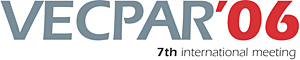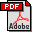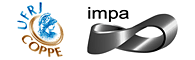vecpar.fe.up.pt/2006 | vecpar2006@fe.up.pt Improving the Numerical Simulation of an Airflow Problem with the BlockCGSI Algorithm Carlos Balsa (FEUP)Michel Dayde (ENSEEIHT/IRIT)Jose Laginha Palma (FEUP)Daniel Ruiz (ENSEEIHT/IRIT)Marianna Braza (IMFT/CNRS) Abstract: Partial spectral information associated with the smallest eigenvalues can be used to improve the solution of consecutive linear systems of equations, namely in the simulation of time-dependent partial differential equations, where at each time step there are several systems with the same spectral properties to be solved. We propose to perform a partial spectral decomposition with the BlockCGSI algorithm in the first time step, and exploit this information to improve the convergence of the Conjugate Gradient algorithm in the solution of the following linear systems. We describe in summary the BlockCGSI algorithm, that is a combination of the block Conjugate Gradient (blockCG) with the Inverse Subspace Iteration. Then, we validate the accelerating strategy in the simulation of the flow around an airplane wing, where the Conjugate Gradient is accelerated through the deflation of the starting residual. Keywords: Large Scale Simulations in all areas of Engineering and Science, Numerical Methods (Linear algebra), Numerical Methods (PDE)Download the fullpaper TopRio de Janeiro | Brazil | 2006 | July | 10 11 12 13